# Implementing Support Vector Machine

## Introduction

We can use the Support Vector Machine algorithm for both regression and classification-based tasks. This blog will take one classification problem and build one SVM model to classify the features accurately.

The Breast Cancer dataset is accessible from Kaggle (from this link). This breast cancer dataset was obtained from the University of Wisconsin Hospitals, Madison, from Dr. William H. Wolberg.

For each cell nucleus, we have five features:

1. Mean Radius: (Float-type) It is the mean of distances from the center to the points on the perimeter.

2. Mean Texture: (Float-type) It is the value of the standard deviation of gray-scale values.

3. Mean Perimeter: (Float-type) It is the circumference of the nucleus cell.

4. Mean Area: (Float-type) It is the total area occupied by the cell.

5. Mean Smoothness: (Float-type) It is the local variation in radius lengths.

Our task is to analyze these features for different records and predict whether a person has breast cancer or not. The target feature is diagnosis (int-type), with two values, 0 or 1, corresponding to benign or malignant cancer cells.

## Step I

The first step in any ML task is to import all the necessary libraries in the notebook.

``````# Basic libraries to manipulate & explore the dataset
import pandas as pd
import numpy as np
import matplotlib.pyplot as plt
%matplotlib inline
import seaborn as sns

# Models from sklearn
from sklearn.model_selection import train_test_split
from sklearn import metrics
from sklearn.svm import SVC``````

## Step II

Exploratory Data Analysis on Breast Cancer Dataset.

### Importing the dataset

``````# I have downloaded and saved the dataset in sample_data folder in Google Colab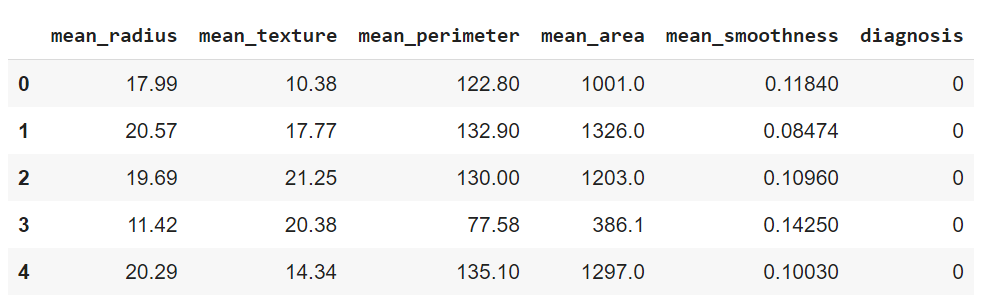### Shape, size, and measures of central tendency analysis

``data.info()``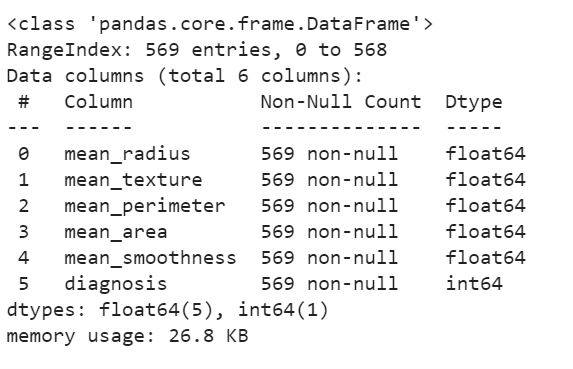``data.describe()``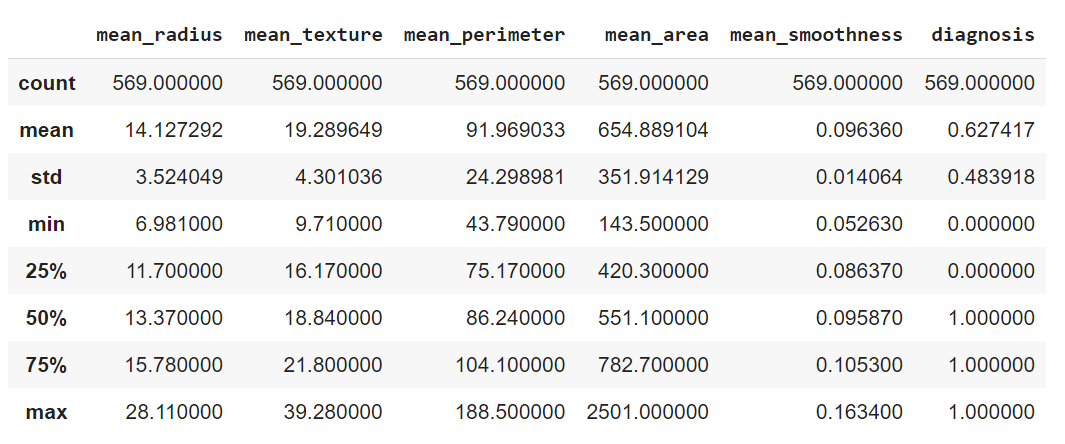### Infographics of the relation between features

``sns.pairplot(data, hue='diagnosis', vars=['mean_radius', 'mean_texture', 'mean_perimeter', 'mean_area', 'mean_smoothness']);``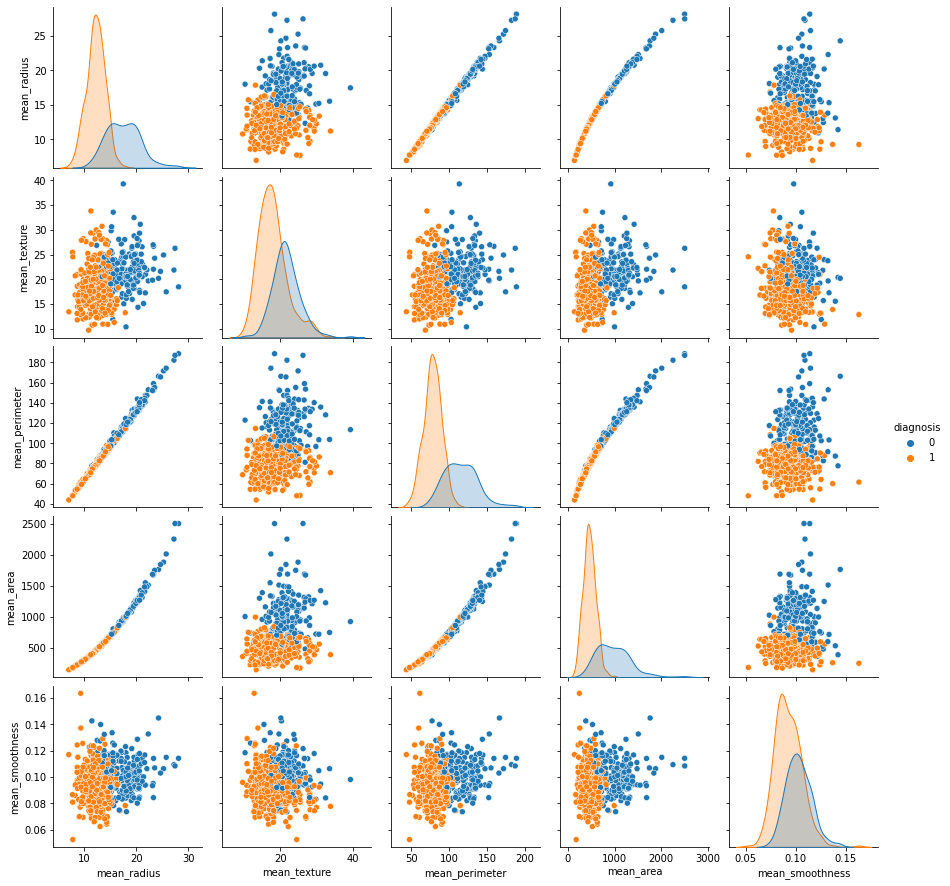``````# Plotting the correlation matrix

plt.figure(figsize=(15, 12))
sns.heatmap(data.corr(), annot=True, cmap='Blues');``````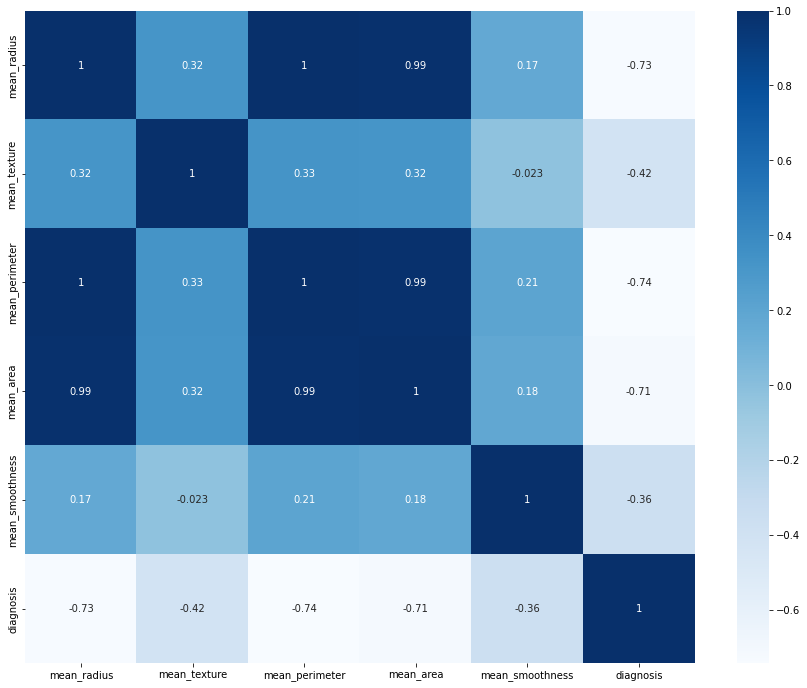### Missing values analysis

``data.isna().sum()``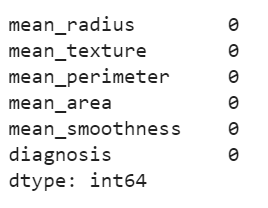### Checking the dataset is balanced or not by plotting target values.

``````graph = sns.countplot(x='diagnosis', data=data);
for p in graph.patches:
graph.annotate('{:.1f}'.format(p.get_height()), (p.get_x()+0.25, p.get_height()+0.01))
``````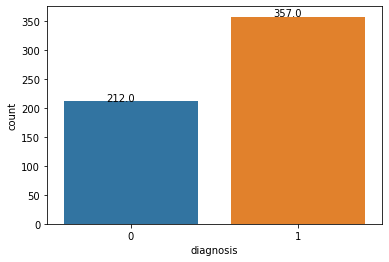## Step III

### Making the dataset suitable for model training.

Splitting the dataset into training and testing - We’ll use the training dataset to train our SVM model for the predictions and the testing dataset to check the accuracy of the predictions.

``````# x contains all the independent features
# y is the target variable

x = data.drop(['diagnosis'], axis=1).values
y = data['diagnosis'].values

#splitting in training and testing
from sklearn.model_selection import train_test_split
x_train, x_test, y_train, y_test = train_test_split(x, y, test_size=0.25, random_state=101)

print("The training data has", x_train.shape, "records.")
print("The testing data has", x_test.shape, "records.")``````## Step IV

### Importing the model and training the model on training dataset

``````from sklearn.svm import SVC
svc_model = SVC()

svc_model.fit(x_train, y_train)
``````

### Generating predictions

``````y_predict = svc_model.predict(x_test)
print(y_predict)``````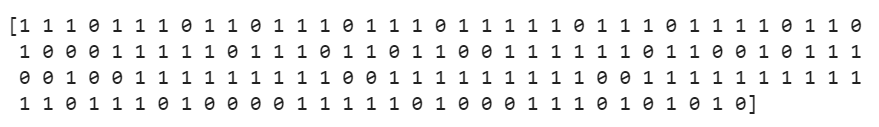## Step V

### Printing classification reports and confusion matrix

``````from sklearn.metrics import classification_report, confusion_matrix

# Confusion Matrix
cm = confusion_matrix(y_test, y_predict)
sns.heatmap(cm, annot=True);``````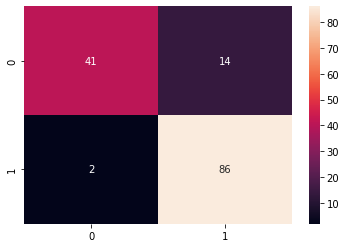``````# Classification Report

cr = classification_report(y_test, y_predict)
print(cr)``````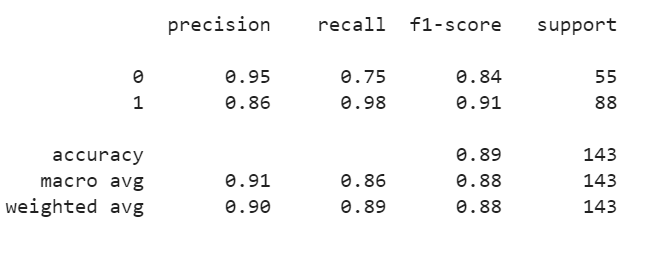## Step VI

### Results: The accuracy of our SVM model is 0.89, which is pretty good.

From the Confusion matrix, we can see the value of True Positives is 41, which means that 41 females have been correctly classified as ‘not having cancer’ and True Negatives is 86, which means that 86 females have correctly been classified as ‘having cancer.’

Our model has predicted that 55 (41 + 14) females don’t have breast cancer; hence the value of False Negative is 14.

The model also classified two females as ‘having cancer,’ but they didn’t have cancer in reality, so False Positive’s value is 2.

1). What are the applications of SVMs?
Support Vector Machine has many use-cases in real-world projects like:

1. Face Detection,
2. Gene Classification,
3. Email Classification,
4. Handwriting Recognition

2). What is the use-case of SVM?

SVM is a supervised machine learning algorithm that can be used for classification or regression problems.

3). What is the standard Kernel Function equation?

The standard kernel function equation is:

## Key Takeaways

Congratulations on making it this far. In this blog, we implemented a Support Vector Machine Algorithm using a Breast Cancer Dataset. Click here, If you want to learn the theoretical aspects of SVM. The kernel is essentially a function to perform calculations even in the higher dimensions in SVM. Learn more about Kernels from this blog.

Check out this link https://www.codingninjas.com/codestudio/library/machine-learning if you are a Machine Learning enthusiast or want to brush up your knowledge with ML blogs.

If you are preparing for the upcoming Campus Placements, then don’t worry. Coding Ninjas has your back. Visit this link for a carefully crafted and designed course on-campus placements and interview preparation.